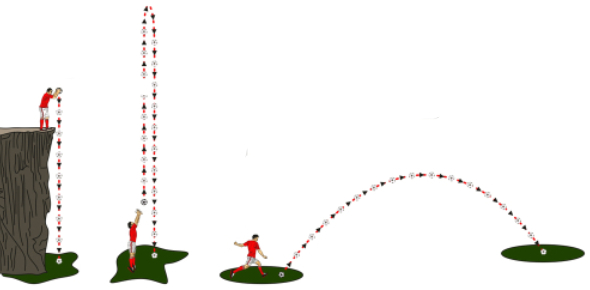# Kinematics Quiz

22 Questions | Attempts: 1364SettingsKinematics is a branch of classical mechanics that describes the motion of points, bodies (objects), and systems of bodies (groups of objects) without considering the mass of each of the forces that caused the motion. It sounds confusing, right? Well, relax and take the quiz to test your knowledge of kinematics.

• 1.
Suppose a car is moving in a straight line and steadily increases its speed.  It moves from 20 m/s to 30 m/s in the first second and from 30 m/s to 40 m/s in the second second.  What is the car's acceleration?
• A.

10 m/s/s

• B.

30 m/s/s

• C.

35 m/s/s

• D.

40 m/s/s

• 2.
An object travels 12 metres in the first second of travel, 12 metres again during the second second of travel and 12 metres again during the third second.  Its acceleration is
• A.

0 m/s/s

• B.

12 m/s/s/

• C.

4 m/s/s

• D.

None of the above

• 3.
If an object has a negative velocity, that means it is
• A.

Slowing down

• B.

Going back towards

• C.

Speeding up while going forward

• D.

All of these

• 4.
A car accelerates at 3 m/s/s, assuming it starts from rest, how much time does it take to accelerate to speed of 45 m/s?
• A.

135 seconds

• B.

90 seconds

• C.

15 seconds

• D.

None of the above

• 5.
A man travels from his home 50m east to the bakery then 20 m south to the butchers, he retraces his steps back to the bakery and stops fora coffee.What is the distance he has traveled and what is his displacement?
• A.

90m, 70, south

• B.

90m east, 0

• C.

70m, 20m north

• D.

90m, 50m east

• 6.
Which of the below shows only vector quantities?
• A.

Distance and displacement

• B.

Displacement, velocity and acceleration

• C.

Speed and velocity

• D.

Speed, velocity and acceleration

• 7.
The school bus speeds up from 20 m/s to 36 m/s in 4 seconds.  What distance does the bus cover in this time frame?
• A.

80 m

• B.

112 m

• C.

64 m

• D.

144 m

• 8.
A brick and a feather are dropped at the same time, from rest in a vacuum (a place where there is no air).  The feather will reach the ground
• A.

Sooner than the brick

• B.

At the same time as the brick

• C.

Later then the brick

• D.

It depends on air resistance

• 9.
The slope of a velocity-time graph tells you
• A.

Maximum velocity

• B.

Acceleration

• C.

Distance traveled

• D.

Where you are

• 10.
The area under a velocity - time graph represents the
• A.

Acceleration

• B.

Distance traveled

• C.

Velocity

• D.

Time

• 11.
What does the position - time graph show from 0 - 15 seconds?
• A.

A person accelerates from rest to 60 m/s in 10 seconds and then travels at a constant velocity for 5 seconds.

• B.

A person climbs up a hill to 60 metres then sits on top of the hill for 5 seconds.

• C.

A person walks with a constant velocity for 60 metres in a positive direction in 10 seconds then remains still for 5 seonds.

• 12.
After 15 seconds select from below which one best describes this position-time graph
• A.

The person turns around and takes 25 seconds to walk 40 m past their original postion before turning around again and taking 15 seconds to return back to where they first started

• B.

The person starts to slow down until they reach -40 m/s then start to speed up again.

• C.

The person climbs down the other side of the hill, it takes them 25 seconds and then they start to climb the next hill

• 13.
Which graph shows an object at rest?
• A.

Graph A

• B.

Graph B

• C.

Graph C

• D.

Graph d

• 14.
Which graph shows constant velocity in a positive direction?
• A.

Graph A

• B.

Graph B

• C.

Graph C

• D.

Graph D

• 15.
The symbol used to represent the acceleration due to gravity is
• 16.
Assuming there is no air resistance, as an object falls freely, its
• A.

Velocity increases

• B.

Acceleration increases

• C.

Both A & B

• D.

None of the above

• 17.
A rock is thrown over a cliff, each second the rock falls
• A.

A larger distance than the second before it

• B.

With the same average speed

• C.

With the same instantaneous speed

• D.

The same distance as the second before

• 18.
Ten seconds after starting from rest, a free falling object will have a velocity of about
• A.

10 m/s

• B.

50 m/s

• C.

100 m/s

• D.

500 m/s

• 19.
Convert 80 km/h into m/s
• A.

0.22 m/s

• B.

22.22 m/s

• C.

222.22 m/s

• D.

0.0022 m/s

• 20.
An abseiler needs to find how high a cliff is.  He drops a small rock over the side, it takes 6 seconds before it hits the ground.  Using 9.8 m/s/s for gravity, how high is the cliff?

## Related TopicsBack to top
×

Wait!
Here's an interesting quiz for you.